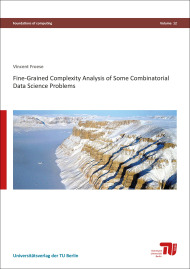# Fine-grained complexity analysis of some combinatorial data science problemsSize: 180 pages
Format: 14,8 x 21,0 cm
Publishing year: 2018
ISBN 978-3-7983-3003-0
11,00

This thesis is concerned with analyzing the computational complexity of NP-hard problems related to data science. For most of the problems considered in this thesis, the computational complexity has not been intensively studied before. We focus on the complexity of computing exact problem solutions and conduct a detailed analysis identifying tractable special cases. To this end, we adopt a parameterized viewpoint in which we spot several parameters which describe properties of a specific problem instance that allow to solve the instance efficiently. We develop specialized algorithms whose running times are polynomial if the corresponding parameter value is constant. We also investigate in which cases the problems remain intractable even for small parameter values. We thereby chart the border between tractability and intractability for some practically motivated problems which yields a better understanding of their computational complexity. In particular, we consider the following problems.
General Position Subset Selection is the problem to select a maximum number of points in general position from a given set of points in the plane. Point sets in general position are well-studied in geometry and play a role in data visualization. We prove several computational hardness results and show how polynomial-time data reduction can be applied to solve the problem if the sought number of points in general position is very small or very large.
The Distinct Vectors problem asks to select a minimum number of columns in a given matrix such that all rows in the selected submatrix are pairwise distinct. This problem is motivated by combinatorial feature selection. We prove a complexity dichotomy with respect to combinations of the minimum and the maximum pairwise Hamming distance of the rows for binary input matrices, thus separating polynomial-time solvable from NP-hard cases.
Co-Clustering is a well-known matrix clustering problem in data mining where the goal is to partition a matrix into homogenous submatrices. We conduct an extensive multivariate complexity analysis revealing several NP-hard and some polynomial-time solvable and fixed-parameter tractable cases.
The generic F-free Editing problem is a graph modification problem in which a given graph has to be modified by a minimum number of edge modifications such that it does not contain any induced subgraph isomorphic to the graph F. We consider three special cases of this problem: The graph clustering problem Cluster Editing with applications in machine learning, the Triangle Deletion problem which is motivated by network cluster analysis, and Feedback Arc Set in Tournaments with applications in rank aggregation. We introduce a new parameterization by the number of edge modifications above a lower bound derived from a packing of induced forbidden subgraphs and show fixed-parameter tractability for all of the three above problems with respect to this parameter. Moreover, we prove several NP-hardness results for other variants of F-free Editing for a constant parameter value.
The problem DTW-Mean is to compute a mean time series of a given sample of time series with respect to the dynamic time warping distance. This is a fundamental problem in time series analysis the complexity of which is unknown. We give an exact exponential-time algorithm for DTW-Mean and prove polynomial-time solvability for the special case of binary time series.